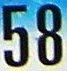## Monday, January 4, 2010

### 58

58 = 2 x 29.

58 has a representation as a sum of two squares: 58 = 32 + 72.

58 is the maximum number of regions into which eight circles divide the plane.

58 is the smallest Smith number with a prime sum of digits. A Smith number is a composite number the sum of whose digits is the sum of the digits of its prime factors: 5 + 8 = 2 + 2 + 9 = 13.

58 is the number of commutative semigroups of order 4.The chemical element cerium has the atomic number 58.

Source: Number Gossip

#### 1 comment:

Jay said...

Still can't explain how fascinating this is. Thumbs up fibonacci!